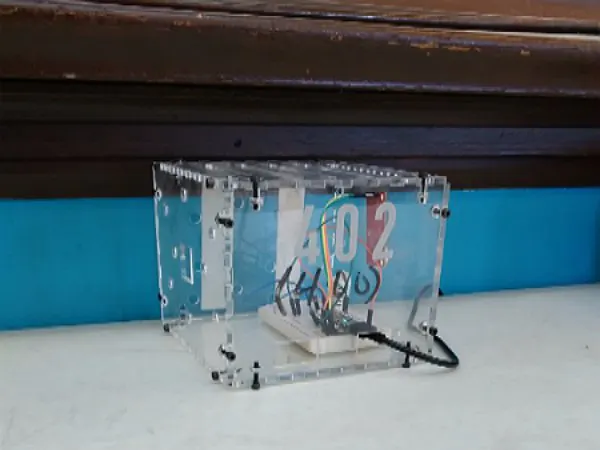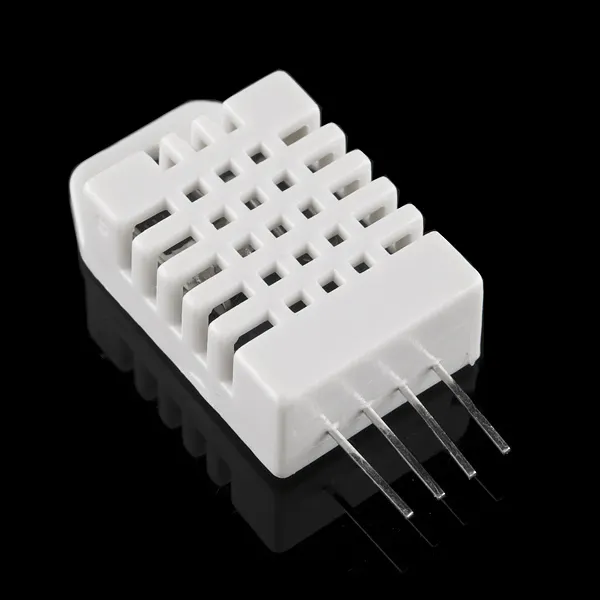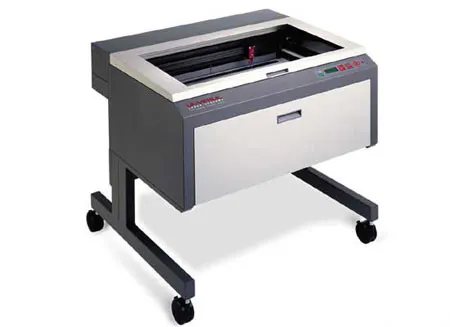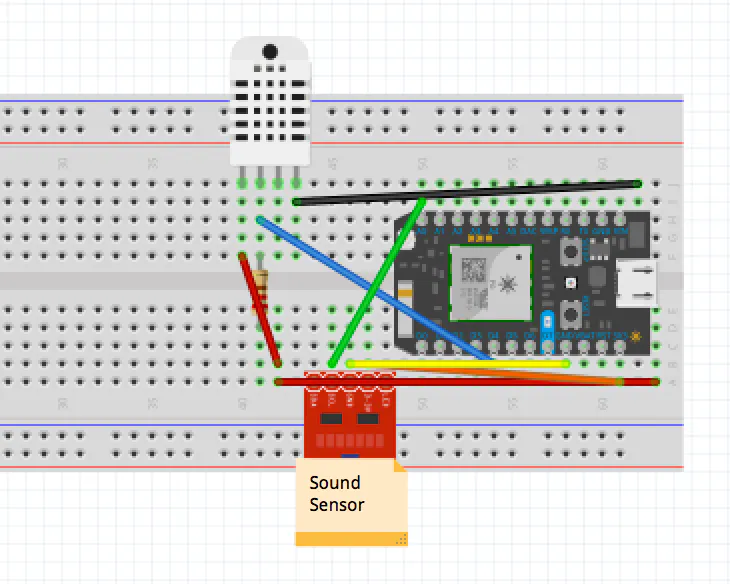Published

# A Pho-ton of Music [Lane of Things]

We collected data using sensors to determine how temperature affects sound level in the orchestra room at Lane Tech.

BeginnerFull instructions provided2 hours250## Things used in this project

### Hardware components

 sparkfun sound
×1Jumper wires (generic) Wires for sound (3) were soldered.
×7Resistor 10k ohm
×1×1DHT22 Temperature Sensor
×1Particle Photon
×1

### Hand tools and fabrication machinesLaser cutter (generic)

## Custom parts and enclosures

### Enclosure

The enclosure is quite simple- even so we decided to use t-slots and acrylic for this.

### Illustrator Screenshot## Schematics

### Fritzing Diagram

The DHT temperature sensor uses 4 wires total. One of these wires must connect to the 3V3 on the photon. For the sound sensor, the necessary outputs are GND, VCC and gate. The gate wire must connect to A0 and the GND output connects to GND on the photon. VCC is the power source, so it must also connect to 3V3 on the photon.## Code

### Sound and Temperature Code

Arduino
```// This #include statement was automatically added by the Particle IDE.

#define DHTPIN 4            // what pin we're connected to
#define DHTTYPE DHT22       // DHT 22 (AM2302)

DHT dht(DHTPIN, DHTTYPE);

double hum;                 // current hum
double temp;                // current temp

const long sample_rate = 50; // time between samples (in miliseconds)
const int array_size = 1200; // 1000/50=20 * 60=1200
int snd_array[array_size] = {};
int snd_max = 0 ;
int snd_min = 4096 ;
double snd_avg = 0;

unsigned long lastSample = 0 ;
unsigned long lastReset = 0 ;

void setup() {
Serial.begin(9600);
pinMode(DHTPIN, INPUT);

Particle.variable("hum", hum);
Particle.variable("temp", temp);
Particle.variable("avg",snd_avg);
Particle.variable("min",snd_min);
Particle.variable("max",snd_max);

Serial.begin(9600);
pinMode(A0, INPUT); // mic AUD connected to Analog pin 0
pinMode(D7, OUTPUT); // flash on-board LED
}

void loop() {
double checkHum = dht.getHumidity();
double checkTemp = dht.getTempFarenheit();

if (checkHum > 0 && checkHum < 100)
hum = checkHum;

if (checkTemp > 32 && checkTemp < 100)
temp = checkTemp;

Serial.println("Temp: " + String(checkTemp));
Serial.println("Hum: " + String(checkHum));

//this will reset the min and max, but keep in mind that those
//large and small values will remain in the array for up to one
//minute depending on the sample_rate
checkReset() ;

delay(3000);
}

{
unsigned long now = millis();

if((now - lastSample) > sample_rate)
{
// Shift all the values right by 1
for(int i = array_size-1; i >= 1; i--)
{
snd_array[i] = snd_array[i-1];
if((snd_array[i] < snd_min) && (snd_array[i] > 0))
{
snd_min = snd_array[i];
}
if(snd_array[i] > snd_max)
{
snd_max = snd_array[i];

}
}

snd_array = value;

// Average of all non-zero elements in the array
double avg_sum = 0;
int size = 0 ;
for (int a=0; a < array_size; a++)
{
if(snd_array[a] > 0)
{
size++ ;
avg_sum += snd_array[a];
}
}
snd_avg = avg_sum / size;

lastSample = now ;
}
}

{
{
digitalWrite(D7, HIGH);
}
else
{
digitalWrite(D7, LOW);
}
}

//check to see if we should reset min and max
void checkReset()
{
unsigned long now = millis();

//if it has been one minute since resetting min and max, reset
if((now - lastReset) >= 60000)
{
snd_max = 0 ;
snd_min = 4095 ;
lastReset = now ;

}
}

//print out the values of avg, min and max to terminal
{
unsigned long now = millis();
Serial.print("Avg: "); Serial.println(snd_avg);
Serial.print("Min: "); Serial.println(snd_min);
Serial.print("Max: "); Serial.println(snd_max);

}
}
```

## Credits

### Emily Stummer

1 project • 1 follower

### Juanairis Castaneda

1 project • 1 follower

### Diego Roman

1 project • 0 followers